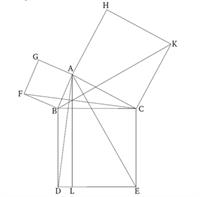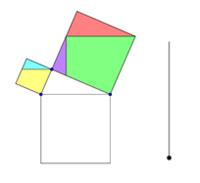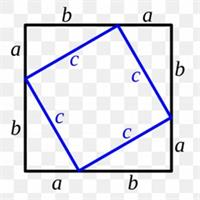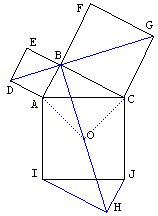# Global AI and Data Science

View Only

## PYTHAGOREAN BROTHERHOOD

Pythagoras was already in ancient times well known for the mathematical achievement of the Pythagorean theorem. Pythagoras had discovered that in a right-angled triangle the square of the hypotenuse is equal to the sum of the squares of the other two sides. In ancient times Pythagoras was also noted for his discovery that music had mathematical foundations. Antique sources that credit Pythagoras as the philosopher who first discovered music intervals also credit him as the inventor of the monochord, a straight rod on which a string and a movable bridge could be used to demonstrate the relationship of musical intervals.The Pythagorean belief that all bodies are composed of numbers and that all properties and causes could be expressed in numbers, served as the basis for a mathematization of science. This mathematization of the physical reality climaxed in the 20th century. The pioneer of physics Werner Heisenberg argued that "this mode of observing nature, which led in part to a true dominion over natural forces and thus contributes decisively to the development of humanity, in an unforeseen manner vindicated the Pythagorean faith".

## ORIGIN OF THE PYTHAGOREAN THEOREM

The Pythagorean theorem was first known in ancient Babylon and Egypt beginning about 1900 B.C. The relationship was shown on the following 4000 year old Babylonian tablet now known as Plimpton 322. However, the relationship was not widely publicized until Pythagoras stated it explicitly.So as per my belief Pythagorean Theorem was discovered when playing with numbers. Following is probably something that they thought of.Archeologists use the Pythagorean in field excavations. When they start a dig, they place a rectangular grid over the site surface. To lay out an accurate grid system, archeologists use the Theorem, X2 + Y2 = Z2. After deciding how long the baselines (X- and Y- axis) should be, the proper length of the diagonal is calculated using the Pythagorean theorem to make sure the quadrant is a rectangle and not a lopsided parallelogram. Corner stakes will then be placed to mark the accurate location of the site.

## PROOF FROM EUCLID’S ELEMENTS

[Proposition 47] In right-angled triangles, the square on the side sub-tending the right-angle is equal to the (sum of the) squares on the sides containing the right-angle.

Let ABC be a right-angled triangle having the angle BAC a right-angle. I say that the square on BC is equal to the sum of the squares on BA and AC.For let the square BDEC have been described on BC, and the squares GB and HC on AB and AC. And let AL have been drawn through point A parallel to either of BD or CE. And let AD and FC have been joined. And since angles BAC and BAG are each right-angles, then two straight-lines AC and AG, not lying on the same side, make the adjacent angles with some straight-line BA, at the point A on it, (whose sum is) equal to two right-angles. Thus, CA is straight-on to AG. So, for the same reasons, BA is also straight-on to AH. And since angle DBC is equal to FBA, for they are both right-angles, let ABC have been added to both. Thus, the whole angle DBA is equal to the angle FBC. Thus, the base AD is equal to the base FC, and the triangle ABD is equal to the triangle FBC. And parallelogram BL is double the area of triangle ABD. For they have the same base, BD, and are between the same parallels, BD and AL. And square GB is double the area of triangle FBC. For again they have the same base, FB, and are between the same parallels, FB and  GC. And the doubles of equal things are  equal to one another. Thus, the parallelogram BL is  also equal to the square GB. So, similarly, AE and BK  being joined, the parallelogram CL can be shown to be equal to the square HC. Thus, the whole square BDEC is equal to the sum of the two squares GB and HC. And the square BDEC is described on BC, and the squares GB and HC on BA and AC respectively. Thus, the square on the side BC is equal to the sum of the squares on the sides BA and AC.

Thus, in right-angled triangles, the square on the side subtending the right-angle is equal to the sum of the squares on the sides surrounding the right-angle. Which is the very thing it was required to show.

PROOFS WITHOUT WORDS## EINSTEIN’S PROOF OF PYTHAGOREAN THEOREM

Einstein in his boyhood got a proof of the Pythagorean Theorem.  Here is the link.

## FEW EXTENSIONS

1. Fermat’s Last Theorem
2. Euclidean Distance
3. Extension to Similar Figures

QUESTION I:

Pythagorean Brotherhood thought of Whole Numbers and Fractions. What is the extension Hippasus thought of that got him killed?

QUESTION II:

Why Absolute Distance is getting more popular than Euclidean Distance now?

#GlobalAIandDataScience
#GlobalDataScience
1 comment
14 views